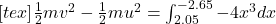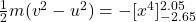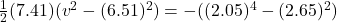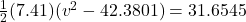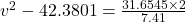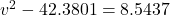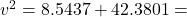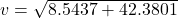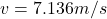## A body with a mass of 7.41 kg moves along the x ‑axis, influenced by a conservative force F ( x ) . → F ( x ) = A x 3 ^ x where A = − 4 N m

Question

A body with a mass of 7.41 kg moves along the x ‑axis, influenced by a conservative force F ( x ) . → F ( x ) = A x 3 ^ x where A = − 4 N m 3 When the body is at position x i = 2.05 m, it is moving with a speed of v i = 6.51 m / s. What is the body’s speed when it is at position x f = − 2.65 m?

in progress 0
5 months 2021-08-09T07:19:45+00:00 1 Answers 2 views 0

7.136 m/s

Explanation:

We are given that

Mass =m=7.41 kg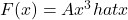Where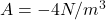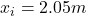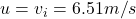We have to find the speed of body when it is position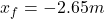According to work energy theorem